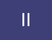cancel
Showing results for
Did you mean:New Member

## Calculated Column within Matrix

I need to calculated already in a Power BI Matrix a column that is Total Value minus column a & b

a & b columns are already defined and whatever is not a & b should go to other category

Current Matrix:

shows a + b = total

a= 3

b=  1

total= 5

I need to generate other column so that it is li

a + b + other = total

a= 3

b=  1

other= 1

total= 5

4 REPLIES 4Community Support

Can you share some sample data in table format and show how the matrix visual looks like currently? For example, what is on rows/columns and what is in values? And what is the matrix expected to be like?

Best Regards,
Community Support Team _ JingNew Member

That was my idea but I keep getting dax formula error. Please, can you explain how?

Many ThanksHelper IHelper I

hi @JorgePerez91 have you tried to create a calculated column in your dataset where you substract the sum of  a+b from Total ?